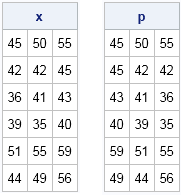Bootstrap methods and permutation tests are popular and powerful nonparametric methods for testing hypotheses and approximating the sampling distribution of a statistic. I have described a SAS/IML implementation of a bootstrap permutation test for matched pairs of data (an alternative to a matched-pair t test) in my paper "Modern Data Analysis for the Practicing Statistician" (Wicklin, 2010, pp 11–14).

The matched-pair permutation test enables you to determine whether the means of the two groups are significantly different. Recently, a SAS user asked how to create a permutation test that compares the means of k groups. An excellent overview of permutation tests in the ANOVA context is provided by M. J. Anderson (2001), who says (p. 628):

Suppose the null hypothesis is true and the groups are not really different (in terms of the measured variable). If this is the case, then the observations are exchangeable between the different groups. That is, the labels that are associated with particular values, identifying them as belonging to a particular group, could be randomly shuffled (permuted) and a new value of [a test statistic]could be obtained.

In a matrix language such as SAS/IML, data is often packed into a matrix with n rows and k columns. (That is, the data are stored in "wide form," as opposed to the "long form" that would be used by the ANOVA or GLM procedures.) One way to implement a permutation test for ANOVA is to apply a permutation to the k elements in each row. The purpose of this article is to provide an efficient way to permute elements within each row of a matrix.

### How to permute elements within rows?

Let's start by defining some data and reading the data into a SAS/IML matrix:

```data test; input t1-t3; datalines; 45 50 55 42 42 45 36 41 43 39 35 40 51 55 59 44 49 56 ;   proc iml; use test; read all into x; close test;```

One approach to permute the elements of each row would be to loop over the rows and apply the RANPERM function to each row. That approach is fine for small data sets, but it is not a vectorized operation. To efficiently permute elements within each row, I will use three facts:

• The RANPERM function can generate n independent random permutations of a set of k elements. Consequently, the RANPERM function can generate a permutation of the column subscripts {1 2 3} for each row.
• The ROW function returns the row number for each element in a matrix. (If you do not have SAS/IML 12.3 or later, I defined the ROW function in a previous blog.)
• A SAS/IML matrices is stored in row-major order. In an n x k matrix, the element with subscript (i,j) is stored in position k(i – 1) + j.

By using these three facts, you can construct a function that independently permutes elements of the rows of a matrix:

```/* independently permute elements of each row of a matrix */ start PermuteWithinRows(m); n = nrow(m); k = ncol(m); j = ranperm(1:k, n); /* each row is permutation of {1 2 ... k} */ matIdx = k*(row(m) - 1) + j; /* matrix position; ROW fcn in 12.3 */ return( shape(m[matIdx], n) ); /* permute elements of m and reshape */ finish;   /* call the function on example data */ call randseed(1234); p = PermuteWithinRows(x); print x p;```The PermuteWithinRows function is very efficient and can be used inside a loop as part of a permutation test. You can also use this technique to implement random permutations in an experimental design.

ShareDistinguished Researcher in Computational Statistics

Rick Wicklin, PhD, is a distinguished researcher in computational statistics at SAS and is a principal developer of SAS/IML software. His areas of expertise include computational statistics, simulation, statistical graphics, and modern methods in statistical data analysis. Rick is author of the books Statistical Programming with SAS/IML Software and Simulating Data with SAS.

1.Ian Wakeling on

I think that matIdx = t(0:(n-1)) @ j(1,k,k) + j; might be a little faster. I wasn't really expecting this, but perhaps it is because you avoid subtracting one from all the elements of row(m)? I tested on a 200*40 matrix and the permutation operation is about 30% quicker.

•I like it. You don't even need to use the Kroneker product operator (@); a simple outer product will do:
matIdx = t(0:(n-1)) * j(1,k,k) + j;

2.Rick,

data test;
input t1-t3;
datalines;
45 50 55
42 42 45
36 41 43
39 35 40
51 55 59
44 49 56
;

proc iml;
use test; read all into x; close test;
ncol=ncol(x);
nrow=nrow(x);
c=colvec(ranperm(1:ncol,nrow));
r=colvec(row(x));
t=colvec(x);
y=full(t||r||c);
print x,y;
quit;

3.Could you help me on one question as below:
now I have a dataset like:
var1 var2 var3
cf555 0.45 1
cg564 0.565 2
vg344 0.23 3

I want to permutate var2 only, if I do the transformation, then i can get
var1 var2 var3
cf555 0.23 1
cg564 0.565 2
vg344 0.45 3

var2 values in line3 change to line1 but the other columns are not changed.

Thanks